Next: Sequential RC Filters Up: Complex Frequencies and the Previous: Complex Frequencies and the

## Poles and Zeros of H

As before, consider expanding the transfer function as the ratio of two polynomials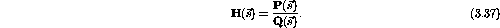If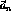are the roots of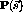and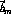are the roots of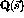we can write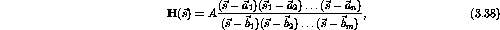where A is a real constant,are zeros of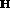andare poles (infinities) of. Knowledge ofanddetermines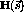everywhere.

Lets now look at our two filter circuits. For a low-pass filter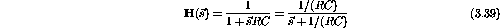and the filter has one pole at -1/(RC). For a high-pass filter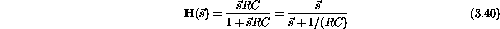and it has one pole at -1/(RC) and one zero at 0. We refer to these two types of filters as single-pole filters.

There is a general rule that there must be at least as many reactive elements as poles. Based on the location of the poles we are able to deduce the general response properties of the filter. We will not do this here.

Example: If a transfer function has poles at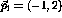and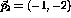and a zero at (0,0), as shown in figure 3.5,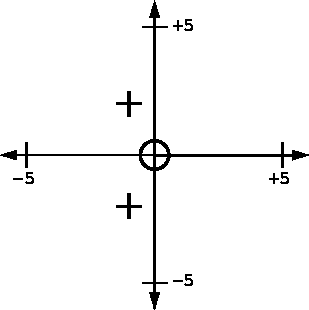Figure 3.5:  Poles and zeros in the complex plane.

1. sketch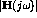on the interval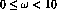.

The transfer function is given by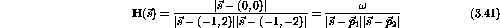Plugging in values forgives the table 3.1.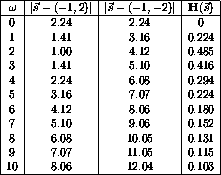Table 3.1:  Numerical values of the transfer function.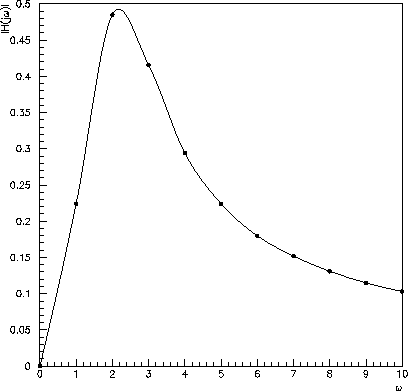Figure 3.6: The transfer function from the table above.

2. If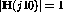, what is the approximate value of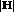at its highest point?

Ifthen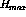at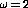is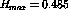. Therefore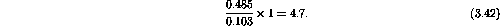Doug Gingrich
Tue Jul 13 16:55:15 EDT 1999